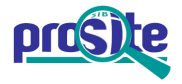Entry: PS01262

 Entry name [info] IF1A Accession [info] PS01262 Entry type [info] PATTERN Date [info] 01-NOV-1997 CREATED; 01-DEC-2004 DATA UPDATE; 02-JUN-2021 INFO UPDATE. PROSITE Doc. [info] PDOC00970

Name and characterization of the entry

 Description [info] Eukaryotic initiation factor 1A signature. Pattern [info] ```[IMVALH]-x-G-x-[GSDENK]-[KRHFW]-x(4)-[CL]-x-D-G-x(2)-[RY]-x(2)-[RH]-[IL]- x-G. ```

Numerical results [info]

Numerical results for UniProtKB/Swiss-Prot release 2021_03 which contains 565'254 sequence entries.

 Total number of hits 57 in 57 different sequences Number of true positive hits 57 in 57 different sequences Number of 'unknown' hits 0 Number of false positive hits 0 Number of false negative sequences 3 Number of 'partial' sequences 0 Precision (true positives / (true positives + false positives)) 100.00 % Recall (true positives / (true positives + false negatives)) 95.00 %

 UniProtKB/Swiss-ProtTrue positive sequences 57 sequences ```IF1A1_HALSA (Q9HN64 ), IF1A1_HALWD (Q18EX1 ), IF1A1_METAC (Q8TSA3 ), ``` ```IF1A1_METBF (Q469J2 ), IF1A1_METMA (Q8PVF1 ), IF1A2_HALSA (Q9HP87 ), IF1A2_HALWD (Q18JF4 ), IF1A2_METAC (Q8TR33 ), IF1A2_METBF (Q469I9 ), IF1A2_METMA (Q8PUJ8 ), IF1A3_METAC (Q8TR31 ), IF1AX_HUMAN (P47813 ), IF1AX_MOUSE (Q8BMJ3 ), IF1AX_PONAB (Q5RA42 ), IF1AY_HUMAN (O14602 ), IF1AY_PANTR (Q6GVM3 ), IF1A_AERPE (P57676 ), IF1A_ARCFU (O29481 ), IF1A_DICDI (Q54YJ6 ), IF1A_HALMA (Q5UZM2 ), IF1A_HALS3 (B0R7D8 ), IF1A_META3 (A6UUK8 ), IF1A_METBU (Q12YN5 ), IF1A_METJA (Q57887 ), IF1A_METKA (Q8TXZ3 ), IF1A_METLZ (A2STQ8 ), IF1A_METM5 (A4FYM5 ), IF1A_METM6 (A9A6C2 ), IF1A_METM7 (A6VJQ7 ), IF1A_METMP (Q6LZM1 ), IF1A_METS5 (A4YEH8 ), IF1A_METTH (O27085 ), IF1A_MOUSE (Q60872 ), IF1A_NATPD (Q3IT14 ), IF1A_ONOVI (P56331 ), IF1A_PICTO (Q6L2G4 ), IF1A_PYRAB (Q9V138 ), IF1A_PYRAE (Q8ZXV9 ), IF1A_PYRFU (Q8U0K5 ), IF1A_PYRHO (O59280 ), IF1A_RABIT (P47814 ), IF1A_RAT (Q6VV72 ), IF1A_SACS2 (Q97W62 ), IF1A_SCHPO (P55877 ), IF1A_SULAC (Q4JA54 ), IF1A_SULIA (C3N0P1 ), IF1A_SULIK (C4KK84 ), IF1A_SULIL (C3MK60 ), IF1A_SULIM (C3MU29 ), IF1A_SULIN (C3NMA0 ), IF1A_SULIY (C3N8M9 ), IF1A_SULTO (Q974Z9 ), IF1A_THEAC (Q03590 ), IF1A_THEKO (Q5JH78 ), IF1A_THEVO (Q979F7 ), IF1A_WHEAT (P47815 ), IF1A_YEAST (P38912 ) ``` » more Retrieve an alignment of UniProtKB/Swiss-Prot true positive hits: [Clustal format, color, condensed view] [Clustal format, color] [Clustal format, plain text] [Fasta format] Retrieve the sequence logo from the alignment UniProtKB/Swiss-ProtFalse negative sequences 3 sequences ```IF1A_DESA1 (B8D5N1 ), IF1A_METST (Q2NEP1 ), YN98_SCHPO (Q9Y803 ) ``` PDB[Detailed view] 28 PDB 1D7Q; 1JT8; 3J80; 3J81; 3JAM; 3JAP; 3JAQ; 3WBK; 3ZJY; 4KZY; 4KZZ; 4MNO; » more